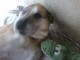Systematic Trading research and development, with a flavour of Trend Following#### January 21st, 2010 · 4 Comments · Backtest

Backtesting mechanical trading systems involves a great deal of optimization: testing and running a system across a myriad of parameters to choose the one(s) that produce the best results.

### But what is “best”?

How do you measure best results, though? Compound Annual Growth Rate (CAGR) is one of the first metric that comes to mind (it is the simplest and most direct one). However consider this:System A produces a greater CAGR – at the cost of much larger variability in the results. I don’t know about you, but “to a point”, I would rather obtain a smoother equity curve and slightly worse CAGR (i.e. choosing System B).

This reasoning leads to a bunch of other metrics and ratio dealing another measure: the variance of the return (Sharpe ratio, Sterling ratio, Sortino ratio, Treynor ratio, Lake ratio, Calmar and MAR ratios, etc.).

In short, there are many ways to quantify how good a system is. One of the first step in the optimization process is to decide on the objective function – which will be used to measure and compare the performance of each system tested in the optimization run (the Sharpe ratio is one such objective function, widely used to compare fund managers).

Ed Seykota coined the term Bliss Function, which suits very aptly: the system that performs best on your set of criteria will give you the most blissful results (wealth increase, peace of mind).

Now consider this:MAR = CAGR / MaxDD (MaxDD = worst drawdown)

System A is very consistent but its return is very unexciting. There might be a threshold where you are willing to accept more risk variance for more reward (i.e. choose to trade system B despite its lower return-to-variability ratio value, like the MAR chosen here). And this is the main point of building an objective/bliss function:

The bliss function should be your own “goodness measure” of a trading system, using your own formula and criteria. Choose a formula that will produce the best results/pick the best systems for you. And you get to be as original as you want!

For the case above, you might decide to complicate the formula of the Bliss function by adding an arbitrary adjustment multiplying function AM to penalise low-returns systems such as:

`Bliss = AM(CAGR) x MAR`

with:
`AM(x<10%) = 0` (ignore any systems producing less than 10%)
`AM(10%<=x<20%) = 0.5` (penalising low-returns systems)
`AM(20%<=x<60%) = 1` (standard value)
`AM(x>=60%) = 1.5` (rewarding high-returns systems)

A continuous function with the same impact would be the logarithmic function.

### Variance != Risk

The shortcut taken by many is to equate return variance with risk. If that is the case, any of your preferred ratio above would be an elegant way to measure risk-adjusted returns.

However, risk and variance are two complete different animals (LTCM had an outstanding Sharpe ratio... until they blew up!)

So, how can you quantify hidden risk? Raw performance return does not allow you to identify it. It really depends on what you consider as Risk.

Hidden risk can be contained in all of these aspects of a strategy that expose you to "risk situations" (think Black Swans) even if they do not materialise.

Time in the Market might be relevant here: all things being equal, you might prefer a strategy in the market 30% of the time rather than 75% - less chance to be hit by this 6-sigma event).

Leverage has a direct influence on the quantitative impact of such event. You might want to introduce measures such as average margin-equity ratio (ME) into your Bliss formula.

Adding the 2 components with arbitrary coefficients the formula would become:

`Bliss = ln(CAGR + 1) x MAR - 0.5 x ME - 0.1 x TimeInMkt%`

So far, we have covered fairly standard metrics for trading systems. But you might want to add your own specific criteria.

One such criteria, which could be interesting to add, is some form of "robustness measure". This will be the subject of a follow-up post.

### In Closing

Coming up with your own bliss function is basically mixing up the various metrics of your trading system (absolute performance, variance, risk and possibly other factors such as robustness) that matter to you. This post tries to highlight the concept and thought process rather than a complete or exact Bliss function.

To expand on the concept of the bliss function, concepts such as walk-forward testing can leverage the bliss function and use it as the objective/selective function.

One can argue that there are as many bliss functions as there are traders. The difficulty lies in finding and formulating its formula...### 4 Comments so far ↓

• […] Jez Liberty of Automated Trading Systems who is posting some very good work lately also  highlights a very subtle concept: 1) the fact that there is no magic metric for measuring performance 2) traders are better off tailoring these metrics to their own personality and preference just like they would a trading system. http://www.automated-trading-system.com/bliss-function-quantify-trading-system-objective/ […]

•cordura21

hey Jez. I think that on the third paragraph “System B produces a greater CAGR” should be “System A…” Cheers, Cord

•Jez Liberty

Hi Cord – Fixed! Thanks for taking the time to let me know..

•Fred

One suggestion that Howard Bandy makes is to 1. run a number of walk forward tests which are optimized on different objective functions such as Calmar ratio, k-ratio, Ulcer Index, etc. 2. print the equity curves and lay them on a table and 3. select the objective function based on the equity curve that you like the most. In a sense this is an elegant way to visually perform the calculation of your own bliss function.